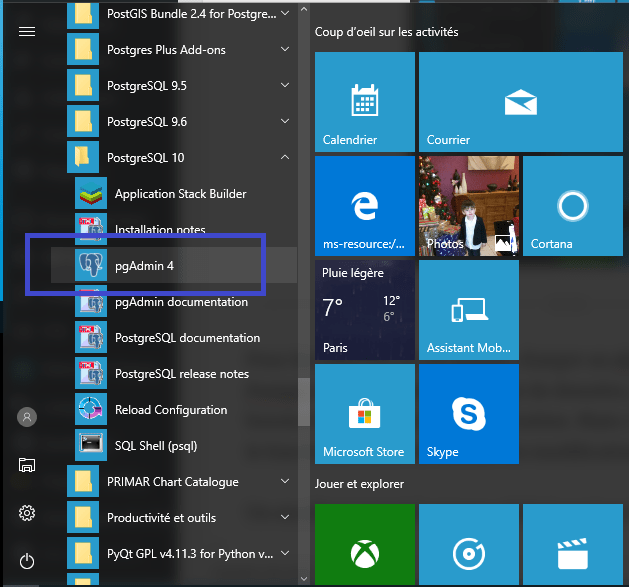## Spatial analysis with SQL: 5- aggregation function (ArcGis Union)

In Article previous we have discussed some SQL functions for spatial processing . In this article we will see a function a little more complicated to put at work: the aggregation function for two layers of polygons. This operation is the one called Union in ArcGis. The purpose of the operation is to obtain a layer with all the intersections of the entities of the two original layers, and, also, all non-intersecting entities.

Let’s use the following example. We have a first layer “rectangles”

A second layer “circles”

That overlaps spatially:

What we want to obtain is a layer containing all the polygons of both layers but creating polygons to overlay areas:

We will proceed by following the same method used in the previous article, i.e. by developing an SQL query including several sub queries.

In the first place, we will develop a query that will build the polygons of the overlapping areas (the intersections) of both layers. These polygons appear in yellow in the next picture.

We will resume the request used in the previous article:

select circle.id as id1, rectangles.id as id2, st_intersection (circle.geom, rectangles.geom ) as the_geom
from circle, rectangles
where st_intersects ( circle.geom, rectangles.geom )
group by circle.id, rectangles.id

You will notice that the select is built not just to retrieve intersected geometries, but also the identifiers of the original entities (circle.id and rectangles.id). Of course, you can recover other attributes, or all by changing this part of the request.

To these polygons, we will add the rest of the areas occupied by the rectangle layer (in yellow in the following picture)

select 0 as id1, rectangles.id as id2, st_difference ( rectangles.geom,

(select multi ST_ (ST_Union (the_geom)) as the_geom from (
select st_intersection (circle.geom, rectangles.geom ) as the_geom
from circle, rectangles
where st_intersects ( circle.geom, rectangles.geom ))
as the_geom)) as the_geom
from rectangles

What we get to do with this request is to extract all the intersection areas (as in the previous query) and convert them in a single multi-polygon. The request removes the difference of this multi- polygon with the layer of rectangles.

Finally, these polygons, we are going to add the rest of the areas occupied by the layer circles to these polygons (yellow in the following image)

We will proceed likewise the rectangles, by using a multi- polygon of intersection areas and calculating the difference with the layer circles:

select circle.id as id1, 0 as id2 , st_difference ( circle.geom , (
select st_multi ( st_union ( the_geom )) as the_geom from (
select st_intersection ( circle.geom, rectangles.geom ) as the_geom
from circle, rectangles
where st_intersects ( circle.geom, rectangles.geom ))
as the_geom )) as the_geom
from circle

The union of these three requests produces the outcome layer we expected :

select *
from
(select circle.id as id1, rectangles.id as id2, st_intersection ( circle.geom, rectangles.geom ) as the_geom
from circle, rectangles
where st_intersects ( circle.geom, rectangles.geom )
group by circle.id, rectangles.id
union all
select 0 as id1, rectangles.id as id2, st_difference ( rectangles.geom , (
select st_multi ( st_union ( the_geom )) as the_geom from (
select st_intersection ( circle.geom, rectangles.geom ) as the_geom
from circle, rectangles
where st_intersects ( circle.geom, rectangles.geom ))
as the_geom )) as the_geom
from rectangles
union all
select circle.id as id1, 0 as id2, st_difference ( circle.geom , (
select st_multi ( st_union ( the_geom )) as the_geom from (
select st_intersection ( circle.geom, rectangles.geom ) as the_geom
from circle, rectangles
where st_intersects ( circle.geom, rectangles.geom ))
as the_geom )) as the_geom
from circle
) as T1

## Replicating a postgresql database in a windows workstation

We will configure a logic   replication of a database table of our linux server in a postgresql installation in a windows workstation. The replication allows us to keep an updated copy of the centralized database in a remote station. This copy will not be used for local updates since it will be read-only. On the other hand, it allows us to work offline and much faster, since the exchanges between the central database and the local replicate contain only the updates of the centralized database. Continue reading “Replicating a postgresql database in a windows workstation”

## How to create a SpatiaLite database with QGis

If you start to feel tired of managing your data as shape files (this format dates back to the eighties!) and you yearn for a genuine database for your projects, most certainly, you will be looking to use PostGis. There is a big gap, and the ‘jump’ is, by no means, an easy one. In this article, we will discuss an alternative solution, the SpatiaLite database. And, believe me, it is much more easier…. Continue reading “How to create a SpatiaLite database with QGis”